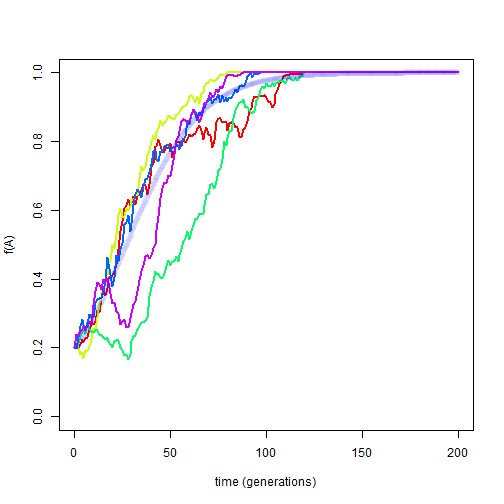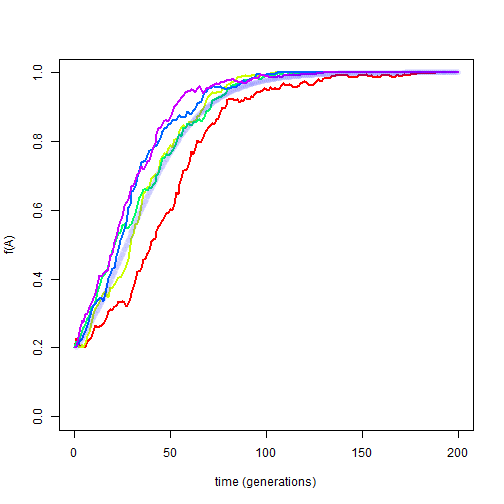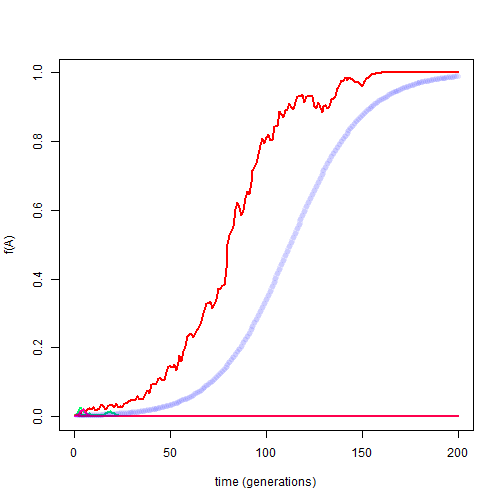## Wednesday, March 7, 2018

### Simulating drift & selection with PopGen

I just added a new function to simulate the combined effects of natural selection & genetic drift for my GitHub package PopGen.

First, here's what the function actually looks like (though it can also be obtained by updating PopGen, as I'll show below):

``````drift.selection<-function(p0=0.5,Ne=100,w=c(1,1,1),ngen=400,nrep=10,
colors=NULL,...){
if(is.null(colors)) colors<-rainbow(nrep)
w<-(w/max(w))[3:1]
gametes<-rep(0,2*Ne)
gametes[1:round(p0*2*Ne)]<-1
gametes<-replicate(nrep,gametes,simplify=FALSE)
p<-lapply(gametes,mean)
for(i in 1:ngen){
genotypes<-lapply(gametes,function(x) matrix(sample(x),
length(x)/2,2))
fitness<-lapply(genotypes,function(x,w) w[rowSums(x)+1],w=w)
selected<-lapply(fitness,function(prob,x)
cbind(sample(x,prob=prob,replace=TRUE),
sample(x,prob=prob,replace=TRUE)),x=Ne)
copy<-replicate(nrep,matrix(sample(1:2,2*Ne,replace=TRUE),
Ne,2),simplify=FALSE)
gametes<-mapply(function(g,s,c) c(diag(g[s[,1],][,c[,1]]),
diag(g[s[,2],][,c[,2]])),
g=genotypes,s=selected,c=copy,SIMPLIFY=FALSE)
for(j in 1:nrep) p[[j]][i+1]<-mean(gametes[[j]])
}
plot(0:ngen,p[],type="l",col=colors,lwd=2,ylim=c(0,1),
xlab="time (generations)",ylab="f(A)")
nulo<-mapply(lines,x=replicate(nrep-1,0:ngen,simplify=FALSE),
y=p[2:nrep],col=colors[2:nrep],lwd=2)
invisible(p)
}
``````

``````library(devtools)
install_github("liamrevell/PopGen")
``````
``````library(PopGen)
``````

First, we can compare drift + selection (using the new function) to selection acting alone:

``````Ne<-200
w<-c(1,0.95,0.9)
drift.selection(p0=0.2,Ne=Ne,w=w,lwd=1,nrep=5,ngen=200)
selection(p0=0.2,w=w,time=200,lwd=6,color=phytools::make.transparent("blue",
``````Clearly we can see the effect of drift, but it is not huge. As we increase Ne this effect should diminish. For example:

``````Ne<-1000
w<-c(1,0.95,0.9)
drift.selection(p0=0.2,Ne=Ne,w=w,lwd=1,nrep=5,ngen=200)
selection(p0=0.2,w=w,time=200,lwd=6,color=phytools::make.transparent("blue",
``````A more realistic scenario, however, might be one in which we start the allele frequency of A at 1/(2Ne). Why? Because this will be the frequency of a new mutation should one arise.

``````Ne<-200
w<-c(1,0.95,0.9)
drift.selection(p0=1/(2*Ne),Ne=Ne,w=w,lwd=1,nrep=20,ngen=200)
selection(p0=1/(2*Ne),w=w,time=200,lwd=6,